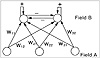# 45.C++ Neural Networks and Fuzzy Logic by Valluru B. Rao M&T Books, IDG Books Worldwide, Inc. ISBN: 1558515526   Pub Date: 06/01/95

### Unsupervised Networks

Unsupervised neural network paradigms to be discussed include:

Hopfield Memory
Bidirectional associative memory
Fuzzy associative memory
Learning vector quantizer
Kohonen self-organizing map
ART1

#### Self-Organization

Unsupervised learning and self-organization are closely related. Unsupervised learning was mentioned in Chapter 1, along with supervised learning. Training in supervised learning takes the form of external exemplars being provided. The network has to compute the correct weights for the connections for neurons in some layer or the other. Self-organization implies unsupervised learning. It was described as a characteristic of a neural network model, ART1, based on adaptive resonance theory (to be covered in Chapter 10). With the winner-take-all criterion, each neuron of field B learns a distinct classification. The winning neuron in a layer, in this case the field B, is the one with the largest activation, and it is the only neuron in that layer that is allowed to fire. Hence, the name winner take all.

Self-organization means self-adaptation of a neural network. Without target outputs, the closest possible response to a given input signal is to be generated. Like inputs will cluster together. The connection weights are modified through different iterations of network operation, and the network capable of self-organizing creates on its own the closest possible set of outputs for the given inputs. This happens in the model in Kohonen’s self-organizing map.

Kohonen’s Linear Vector Quantizer (LVQ) described briefly below is later extended as a self-organizing feature map. Self-organization is also learning, but without supervision; it is a case of self-training. Kohonen’s topology preserving maps illustrate self-organization by a neural network. In these cases, certain subsets of output neurons respond to certain subareas of the inputs, so that the firing within one subset of neurons indicates the presence of the corresponding subarea of the input. This is a useful paradigm in applications such as speech recognition. The winner-take-all strategy used in ART1 also facilitates self-organization.

### Learning Vector Quantizer

Suppose the goal is the classification of input vectors. Kohonen’s Vector Quantization is a method in which you first gather a finite number of vectors of the dimension of your input vector. Kohonen calls these codebook vectors. You then assign groups of these codebook vectors to the different classes under the classification you want to achieve. In other words, you make a correspondence between the codebook vectors and classes, or, partition the set of codebook vectors by classes in your classification.

Now examine each input vector for its distance from each codebook vector, and find the nearest or closest codebook vector to it. You identify the input vector with the class to which the codebook vector belongs.

Codebook vectors are updated during training, according to some algorithm. Such an algorithm strives to achieve two things: (1), a codebook vector closest to the input vector is brought even closer to it; and (two), a codebook vector indicating a different class is made more distant from the input vector.

For example, suppose (2, 6) is an input vector, and (3, 10) and (4, 9) are a pair of codebook vectors assigned to different classes. You identify (2, 6) with the class to which (4, 9) belongs, since (4, 9) with a distance of [radic]13 is closer to it than (3, 10) whose distance from (2, 6) is [radic]17. If you add 1 to each component of (3, 10) and subtract 1 from each component of (4, 9), the new distances of these from (2, 6) are [radic]29 and [radic]5, respectively. This shows that (3, 10) when changed to (4, 11) becomes more distant from your input vector than before the change, and (4, 9) is changed to (3, 8), which is a bit closer to (2, 6) than (4, 9) is.

Training continues until all input vectors are classified. You obtain a stage where the classification for each input vector remains the same as in the previous cycle of training. This is a process of self-organization.

The Learning Vector Quantizer (LVQ) of Kohonen is a self-organizing network. It classifies input vectors on the basis of a set of stored or reference vectors. The B field neurons are also called grandmother cells, each of which represents a specific class in the reference vector set. Either supervised or unsupervised learning can be used with this network. (See Figure 6.2.)Figure 6.2  Layout for Learning Vector Quantizer.C++ Neural Networks and Fuzzy Logic
ISBN: 1558515526
EAN: 2147483647
Year: 1995
Pages: 139

Similar book on Amazon